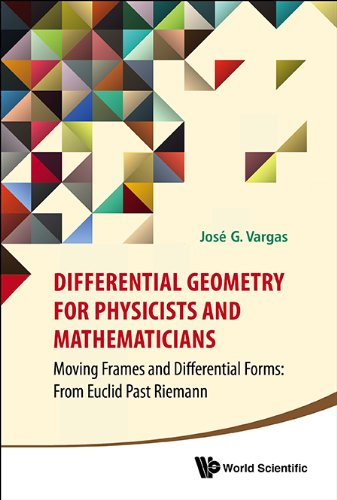# Differential Geometry for Physicists and Mathematicians

Description

This is a book that the author wishes had been available to him when he was student. It reflects his interest in knowing (like expert mathematicians) the most relevant mathematics for theoretical physics, but in the style of physicists. This means that one is not facing the study of a collection of definitions, remarks, theorems, corollaries, lemmas, etc. but a narrative — almost like a story being told — that does not impede sophistication and deep results.

It covers differential geometry far beyond what general relativists perceive they need to know. And it introduces readers to other areas of mathematics that are of interest to physicists and mathematicians, but are largely overlooked. Among these is Clifford Algebra and its uses in conjunction with differential forms and moving frames. It opens new research vistas that expand the subject matter.

In an appendix on the classical theory of curves and surfaces, the author slashes not only the main proofs of the traditional approach, which uses vector calculus, but even existing treatments that also use differential forms for the same purpose.

Readership: Physicists and mathematicians working on differential geometry.

Part I Introduction
Chapter 1 Orientations
Part II Tools

Chapter 2 Differential Forms
Chapter 3 Vector Spaces And Tensor Products
Chapter 4 Exterior Differentiation

Part III Two Klein Geometries
Chapter 5 Affine Klein Geometry
Chapter 6 Euclidean Klein Geometry

Part Iv Cartan Connections
Chapter 7 Generalized Geometry Made Simple
Chapter 8 Affine Connections
Chapter 9 Euclidean Connections
Chapter 10 Riemannian Spaces And Pseudo-Spaces

Part V The Future?
Chapter 11 Extensions Of Cartan
Chapter 12 Understand The Past To Imagine The Future
Chapter 13 A Book Of Farewells

Chapter Appendix A: Geometry Of Curves And Surfaces
Chapter Appendix B: “Biographies” (“Publi” Graphies)
Chapter Appendix C: Publications By The Author